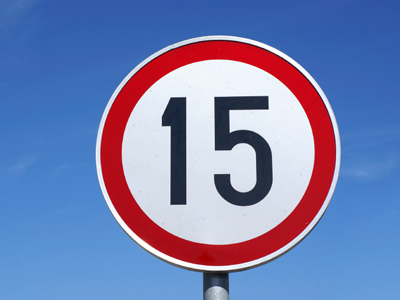15 squared is 225.

# Properties of Numbers (Year 6)

In KS2 Maths children will come to understand some of the properties of numbers. In Year Six children should fully understand multiples and factors. They should also be familiar with square numbers and will also know what prime numbers are.

The properties of numbers are things like square numbers, factors, multiples and prime numbers. Knowing these properties of numbers is all about understanding the relationships between them. For instance, what is a square number or a prime number? Well, a square number is the product when a number is multiplied by itself and a prime number is one whose only factors are itself and 1.

How much do you know about the properties of numbers? Find out in this quiz for 10-11 year olds.

1.
How can we recognise if a number is divisible by 8?
A number is divisible by 8 if it is a square number
A number is divisible by 8 if it ends in 2 or 4
A number is divisible by 8 if the last two digits are divisible by 8
A number is divisible by 8 if the last three digits are divisible by 8
Or if half the number is divisible by 4
2.
How can we recognise if a number is divisible by 3?
A number is divisible by 3 if it is an odd number
A number is divisible by 3 if it ends in 3
A number is divisible by 3 if the sum of its digits is divisible by 3
A number is divisible by 3 if it is a square number
642 is divisible by 3 because 6 + 4 + 2 = 12; 12 is divisible by 3
3.
Which are the factors of 24?
1, 2, 3, 4, 6, 8, 12, 24
2, 3, 4, 6, 8, 12
1, 2, 3, 4, 5, 8, 12, 24
1, 2, 4, 6, 12, 24
Remember that every number is a factor of itself as it is itself multiplied by 1
4.
How can we recognise if a number is divisible by 25?
A number is divisible by 25 if it ends in 00, 25, 50 or 75
A number is divisible by 25 if it is divisible by 8
A number is divisible by 25 if the last two digits are divisible by 8
A number is divisible by 25 if it is odd and divisible by 3
Despite it being a relatively large number, the 25 times table is quite easy to learn
5.
How can we recognise if a number is divisible by 9?
A number is divisible by 9 if it is an odd number
A number is divisible by 9 if the sum of its digits is divisible by 9
A number is divisible by 9 if the last two digits are divisible by 4
A number is divisible by 9 if the sum of its digits total 3
468 is divisible by 9 because 4 + 6 + 8 = 18 and 18 = 2 x 9
6.
What is a prime number?
A number that is only divisible by 1 and itself
A number that cannot be squared
A whole number that divides into another whole number
An odd number that is divisible by 3
For a number to be prime it must have 2 different factors, therefore 1 is not counted as a prime number
7.
Which is the smallest common factor of 6 and 15?
2
3
5
6
3 is in fact the only common factor of 6 and 15
8.
What is 15 squared?
150
175
225
250
One way to work this out is to multiply 15 by 10 (150), then half it (75) and add the two answers together (150 + 75 = 225)
9.
How can we recognise if a number is divisible by 6?
A number is divisible by 6 if it is odd and divisible by 3
A number is divisible by 6 if it is even and divisible by 3
A number is divisible by 6 if the sum of its digits total 3
A number is divisible by 6 if it is even and divisible by 2
96 is divisible by 6 because it is also divisible by 3 and it is even
10.
What is the next prime number after 7?
9
11
13
27
Here are all the prime numbers between 1 and 50
2 - 3 - 5 - 7 - 11 - 13 - 17 - 19 - 23 - 29 - 31 - 37 - 41 - 43 - 47